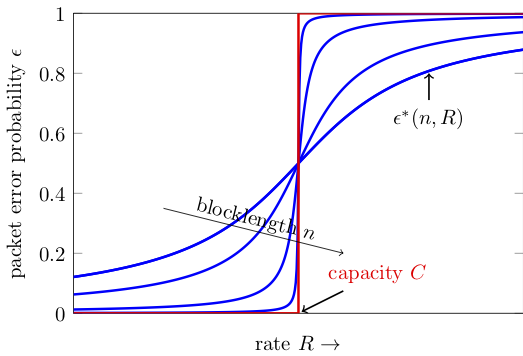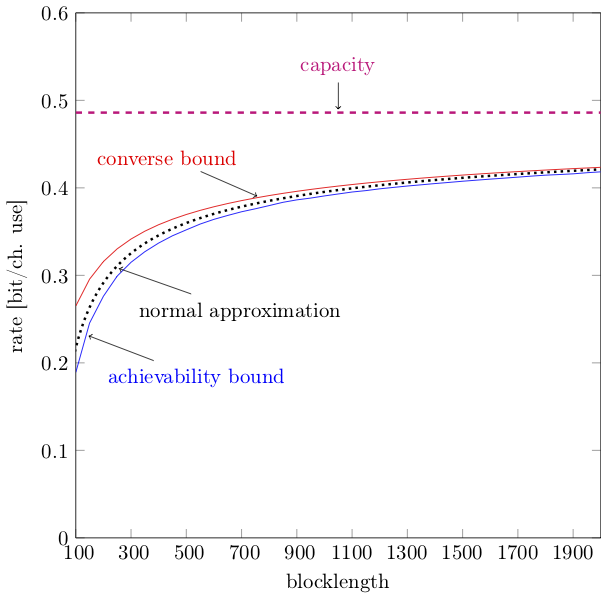# Designing URLLC via nonasymptotic information theory–part 2

An updated and expanded version of these notes can be found

We will start our tour through finite-blocklength information theory by analyzing one of the simplest, yet practically relevant, point-to-point communication channel: the discrete-time binary-input AWGN channel. The input-output relation of such a channel is

$y_j=\sqrt{\rho}x_j + w_j, \quad j=1,\dots, n \label{eq:io}$

Here, $x_k$ is the channel input, which we assume to belong to the set $\{-1,1\}$ whereas $\{w_j\}$ is the discrete-time AWGN process. We assume it to be stationary memoryless and that each of its samples has zero mean and unit variance. Hence, $\rho$ can be interpreted as the signal-to-noise ratio (SNR). Throughout, we shall assume that, to transmit an information message, i.e., a set of $k$ information bits, we are allowed to use the bi-AWGN channel $n$ times.

Note that $n$ is closely related to the communication latency. Indeed, assume that to carry each binary symbol $x_k$ we use a continuous-time signal of bandwidth $W$ Hz. Then using the channel $n$ times to transmit our message of $k$ bits entails a delay of $n/W$ seconds. The actual overall delay may be larger if we also include queuing, propagation, and decoding delays. But in a first approximation, we will neglect these additional sources of delay and take $n$ as a proxy for latency. This is not a bad assumption for short-distance communications. In 4G for example, the smallest packet size has a duration of 0.5 milliseconds, whereas the propagation delay to cover a distance of 180 meters is just 30 microseconds.

So if we are interested in low latency, we should choose $n$ as small as possible. But there is a tradeoff between latency and reliability. Indeed, it is intuitively clear that the larger the number of channel uses $n$ we are allowed to occupy for the transmissions of our $k$ bits is, the more reliable the transmission will be. Let us then denote by $\epsilon$ the error probability. We are interested in the following question.

What is the smaller $n$ for which the $k$ information bits can be decoded correctly with probability $1-\epsilon$ or larger?

Equivalently, we may try to find the largest number of bits $k$ that we can transmit with reliability $1-\epsilon$ or larger using the channel $n$ times, or the smallest probability of error $\epsilon$ that can be achieved for given $k$ and $n$.

What we will do next is to introduce some notation that will help us characterizing these quantities, and also illustrate why standard asymptotic information theory does not provide satisfactory answers.

How do we transmit the $k$ information bits using $n$ channel uses and how do we recover the $k$ bits from the $n$ received samples $y^n=\{y_1,\dots,y_n\}$? We use a channel code, i.e., an encoder that maps the $k$ bits into the $n$ channel uses, and a decoder that provides an estimate of the $k$ information bits from $y^n$.

Here is a more formal definition:

A $(k,n,\epsilon)$-code for the bi-AWGN channel with a given SNR $\rho$ consists of (i) an encoder $f:\{1,2,\dots, 2^k\}\mapsto \{-1,1\}^n$ that maps the information message $j \in \{1,2,\dots, 2^k\}$ into $n$ BPSK symbols. We will refer to the set of all $n$-dimensional strings produced by the encoder as codebook. Furthermore, we will call each of the string a codewords and $n$ the blocklength. (ii) A decoder $g:\mathbb{R}^n\mapsto \{1,2,\dots, 2^k\}$ that maps the received sequence $y^n \in \mathbb{R}^n$ into a message $\widehat{\jmath}\in \{1,2,\dots, 2^k\}$, or, possibly, it declares an error. This decoder satisfies the error-probability constraint $\mathrm{Pr}\bigl\{\widehat{\jmath}\neq j\bigr\}\leq \epsilon$.

Note that $\epsilon$ should be interpreted as a packet/frame error probability and not as a bit error probability. Indeed, a difference in a single bit between the binary representation of $\jmath$ and $\widehat{\jmath}$ causes an error, according to our definition.

In a nutshell, finite-blocklength information theory allows us to find, for a given SNR, the triplets $(k,n,\epsilon)$ for which a $(k,n,\epsilon)$-code can be found and the triplets $(k,n,\epsilon)$ for which no code exists.

Note that doing so is not trivial, since an exhaustive search among all codes is hopeless. Indeed, even if we fix the decoding rule to, for example, maximum likelihood, for a given choice of $k$ and $n$, one would need to go through $\binom{2^n}{2^k}$ codes.

Before exploring how and in which sense a characterization of the triplets $(k,n,\epsilon)$ can be performed, let us first see what we can do by using the notion of channel capacity introduced by Shannon in his 1948 groundbreaking paper 1.

Let us denote by $k^\star(n,\epsilon)$ the largest number of bits we can transmit using $n$ channel uses with error probability not exceeding $\epsilon$ and by $R^\star(n,\epsilon)=k^\star(n,\epsilon)/n$ the corresponding maximum coding rate, measured in bits per channel use.

Also for a given rate $R=k/n$, let us denote by $\epsilon^\star(n,R)$ the smallest error probability achievable using $n$ channel uses and a code of rate $R$.

Since we cannot go through all codes, none of these quantities can be actually computed for the parameter values that are of interest in typical wireless communication scenarios. Shannon’s capacity provides an asymptotic characterization of these quantities.

Specifically, Shannon’s capacity $C$ is the largest communication rate at which we can transfer information messages over the channel \eqref{eq:io} with arbitrarily small error probability for sufficiently large blocklength.

Mathematically,

\begin{equation} C=\lim_{\epsilon \to 0} \lim_{n\to \infty} R^\star(n,\epsilon). \end{equation}

• I’ve been a bit sloppy with the definition of $C$. One should in general worry about whether the limits exist. They do for the memoryless channel under analysis here.
• It is important to take the limits in this precise order. If we were to invert the order of the limits, we would get $C=0$ for the bi-AWGN channel \eqref{eq:io}: a not very insightful result.
• For the channel in \eqref{eq:io} (and for many other channels of interest in wireless communications), the first limit $\epsilon\to 0$ is actually superfluous. We get the same result for every $\epsilon$ in the interval $(0,1)$ as a consequence of the so-called strong converse.

One way to explain the relation between capacity and finite-blocklength metrics is through the following figure, which artistically illustrates $\epsilon^\star(n,R)$ as a function for $R$ for various values of $n$.Although we do not know how to compute $\epsilon^\star(n,R)$, Shannon was able to show that this function converges to a step function centered at $C$ in the limit $n\to\infty$. When $R<C$, one can achieve arbitrarily low $\epsilon$ by choosing $n$ sufficiently large. If however $R>C$ the packet error probability goes to $1$ as we increase $n$.

We know from Shannon’s channel coding theorem that, for stationary memoryless channels like the binary input AWGN channel \eqref{eq:io}, the channel capacity $C$ is

$C=\max_{P_X} I(X;Y) \label{eq:capacity}$

where $P_X$ denotes the probability distribution on the input $X$ and $I(X;Y)$ is the mutual information

$I(X;Y)= \mathbb{E}\left[\log\frac{P_{Y|X}(Y|X)}{P_Y(Y)}\right]. \label{eq:mutual_info}$

Here, the expectation is with respect to the joint distribution of $(X,Y)$. Furthermore, $P_{Y |X}$ denotes the channel law

$P_{Y | X} (y |x)=\frac{1}{\sqrt{2\pi}}\exp\left(- \frac{(y-\sqrt{\rho}x)^2}{2} \right)$

and $P_Y$ is the distribution on the channel output $Y$ induced by $P_X$ through $P_{Y | X}$. Specifically, $P_Y(y)=\mathrm{E}\left[P_{Y|X}(y|\bar{X})\right]$ where $\bar{X}\sim P_X$ and independent of $X$.

A remark on notation is in order. It will be convenient from now on to distinguish between deterministic and random quantities. I will use uppercase letter such as $X$ to denote random quantities , and lowercase letters such as $x$ to denote their realizations.

It will also be convenient to give a name to the loglikelihood ratio in the argument of the expectation that defines mutual information in \eqref{eq:mutual_info}. It is usually called information density and denoted by $i(x;y)$. Hence, $I(X;Y)=\mathrm{E}[i(X;Y)]$.

For the binary-input AWGN channel, one can show that the uniform distribution over $\{-1,1\}$ achieves the maximum in \eqref{eq:capacity}. The induced output distribution $P_Y$ is a Gaussian mixture $P_Y= \frac{1}{2}\mathcal{N}(-\sqrt{\rho},1)+\frac{1}{2}\mathcal{N}(\sqrt{\rho},1)$ and the information density can be readily computed as

$i(x;y) = \log 2 - \log\left(1+ \exp(-2xy\sqrt{\rho})\right).$

Finally, the channel capacity $C$ is given by

\begin{equation} C=\frac{1}{\sqrt{2\pi}}\int e^{-z^2/2} \Bigl(\log(2)-\log\bigl(1+e^{-2\rho-2z\sqrt{\rho}}\bigr) \Bigr)\, \mathrm{d}z. \end{equation}

Computing capacity takes only few lines of matlab code

f = @(z) exp(-z.^2/2)/sqrt(2*pi) .* (log2(2) - log2(1+exp(-2*rho-2*sqrt(rho)*z)));
Zmin = -9; Zmax = 9;


This code is part of the routine biawgn_stats.m available as part of the SPECTRE toolbox. I will talk about toolbox in later posts; nevertheless, it may be good to have a look at it already now.

To summarize, the capacity of the bi-AWGN is relatively easy to compute, but gives us only the following asymptotic answer to the question about which triplets $(k,n,\epsilon)$ are achievable:

All triplets $(nR,n,\epsilon)$ are achievable for sufficiently large $n$, provided that $R<C$.

As we shall see, finite-blocklength information theory will give us a much more precise characterization. The following numerical example gives a flavor of the results we will derive in the next posts. Fix $\rho=0$ dB. For this SNR value, the capacity of the bi-AWGN channel is about $0.486$ bit/channel use. In the figure below, I plotted two bounds and an approximation on $R^\star(k,n)$ as a function of the blocklength $n$, for the case $\epsilon=10^{-4}$, obtained through finite-blocklength information theory.One can see that the bounds and the approximation allow one to identify accurately, for a fixed $\epsilon$, the pairs $(k,n)$ that are achievable. Such a precise characterization is indispensable for an optimum design of URLLC.

Go to back to part 1 or move on to part 3

1. C. E. Shannon, “A mathematical theory of communication,” Bell Syst. Tech. J., vol. 27, pp. 379–423 and 623–656, July and October 1948.

Tags:

Categories:

Updated: